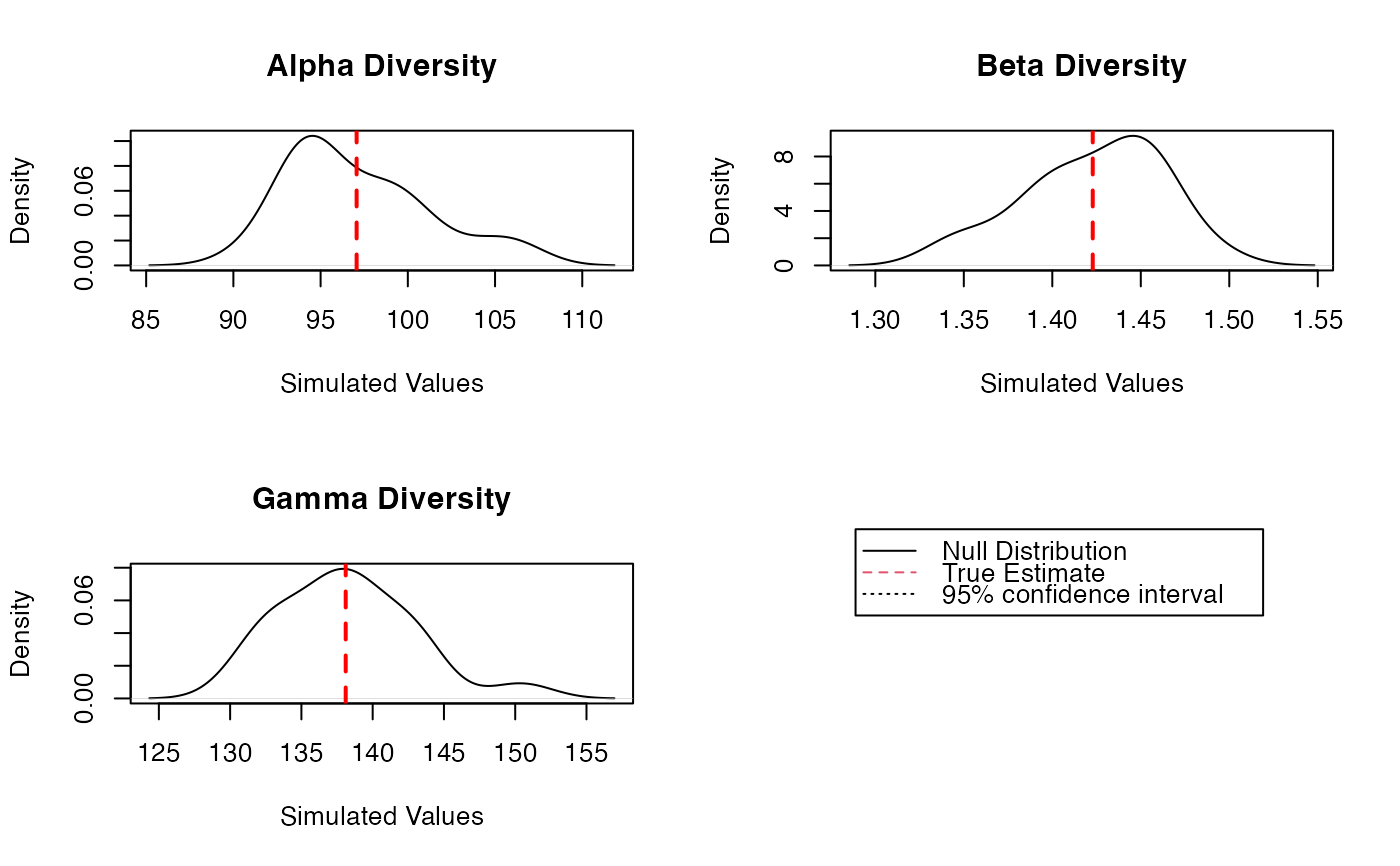Estimates diversity of a metacommunity.

DivEst(q = 0, MC, Biased = TRUE, Correction = "Best", Tree = NULL,
Normalize = TRUE, Z = NULL, Simulations = 100,
ShowProgressBar = TRUE, CheckArguments = TRUE)
is.DivEst(x)
# S3 method for DivEst
plot(x, ..., main = NULL, Which = "All",
Quantiles = c(0.025, 0.975), colValue = "red", lwdValue = 2, ltyValue = 2,
colQuantiles = "black", lwdQuantiles = 1, ltyQuantiles = 2)
# S3 method for DivEst
autoplot(object, ..., main = NULL, Which = "All",
labels = NULL, font.label = list(size=11, face="plain"),
Quantiles = c(0.025, 0.975), colValue = "red",
colQuantiles = "black", ltyQuantiles = 2)
# S3 method for DivEst
summary(object, ...)

## Arguments

q

A number: the order of diversity.

MC

A MetaCommunity object.

Biased

Logical; if FALSE, a bias correction is appplied.

Correction

A string containing one of the possible corrections. The correction must be accepted by DivPart. "Best" is the default value.

Tree

An object of class hclust, phylo, phylog or PPtree. The tree must be ultrametric.

Normalize

If TRUE (default), diversity is not affected by the height of the tree..
If FALSE, diversity is proportional to the height of the tree.

Z

A relatedness matrix, i.e. a square matrix whose terms are all positive, strictly positive on the diagonal. Generally, the matrix is a similarity matrix, i.e. the diagonal terms equal 1 and other terms are between 0 and 1.

Simulations

The number of simulations to build confidence intervals.

ShowProgressBar

If TRUE (default), a progress bar is shown.

CheckArguments

Logical; if TRUE, the function arguments are verified. Should be set to FALSE to save time when the arguments have been checked elsewhere.

x

An object to be tested or plotted.

main

The title of the plot.

Which

May be "Alpha", "Beta" or "Gamma" to respectively plot the metacommunity's alpha, beta or gamma diversity. If "All" (default), all three plots are shown.

labels

Vector of labels to be added to multiple plots. "auto" is the same as c("a", "b", "c", "d)".

font.label

A list of arguments to customize labels. See ggarrange.

object

A MCdiversity object to be summarized or plotted.

Quantiles

A vector containing the quantiles of interest.

colValue

The color of the line representing the real value on the plot.

lwdValue

The width of the line representing the real value on the plot.

ltyValue

The line type of the line representing the real value on the plot.

colQuantiles

The color of the lines representing the quantiles on the plot.

lwdQuantiles

The width of the lines representing the quantiles on the plot.

ltyQuantiles

The line type of the lines representing the quantiles on the plot.

...

Additional arguments to be passed to the generic methods.

## Details

Divest estimates the diversity of the metacommunity and partitions it into alpha and beta components.

If Tree is provided, the phylogenetic diversity is calculated else if Z is not NULL, then similarity-based entropy is calculated.

Bootstrap confidence intervals are calculated by drawing simulated communities from a multinomial distribution following the observed frequencies (Marcon et al, 2012; 2014).

## Value

A Divest object which is a DivPart object with an additional item in its list:

SimulatedDiversity

A matrix containing the simulated values of alpha, beta and gamma diversity.

Divest objects can be summarized and plotted.

## Author

Eric Marcon <Eric.Marcon@agroparistech.fr>, Bruno Herault <Bruno.Herault@cirad.fr>

DivPart

## Examples

# Load Paracou data (number of trees per species in two 1-ha plot of a tropical forest)
data(Paracou618)
# Estimate Shannon diversity.
Estimation <- DivEst(q = 1, Paracou618.MC, Biased = FALSE, Correction = "UnveilJ",
Simulations = 20)
#>
plot(Estimation)
#> Warning: NAs introduced by coercion
#> Warning: NAs introduced by coercion
#> Warning: NAs introduced by coercion
#> Warning: NAs introduced by coercion
#> Warning: NAs introduced by coercion
#> Warning: NAs introduced by coercionsummary(Estimation)
#> Diversity partitioning of order 1 of MetaCommunity Paracou618.MC
#>  with correction: UnveilJ
#> Alpha diversity of communities:
#>     P006     P018
#>  83.7268 118.2713
#> Total alpha diversity of the communities:
#>  97.06467
#> Beta diversity of the communities:
#>  UnveilJ
#> 1.422843
#> Gamma diversity of the metacommunity:
#>  UnveilJ
#> 138.1078
#> Quantiles of simulations (alpha, beta and gamma diversity):
#>        0%        1%      2.5%        5%       10%       25%       50%       75%
#>  90.58253  90.83297  91.20863  91.83474  92.32861  94.29321  96.87478  99.65053
#>       90%       95%     97.5%       99%      100%
#> 101.73709 102.36111 103.07545 103.50406 103.78980
#>       0%       1%     2.5%       5%      10%      25%      50%      75%
#> 1.377156 1.377222 1.377320 1.377483 1.378761 1.403860 1.419430 1.439966
#>      90%      95%    97.5%      99%     100%
#> 1.467971 1.478549 1.488731 1.494840 1.498913
#>       0%       1%     2.5%       5%      10%      25%      50%      75%
#> 129.9730 130.0242 130.1010 130.2290 130.9031 133.6503 139.8732 141.8658
#>      90%      95%    97.5%      99%     100%
#> 144.0250 145.1951 146.3578 147.0555 147.5206